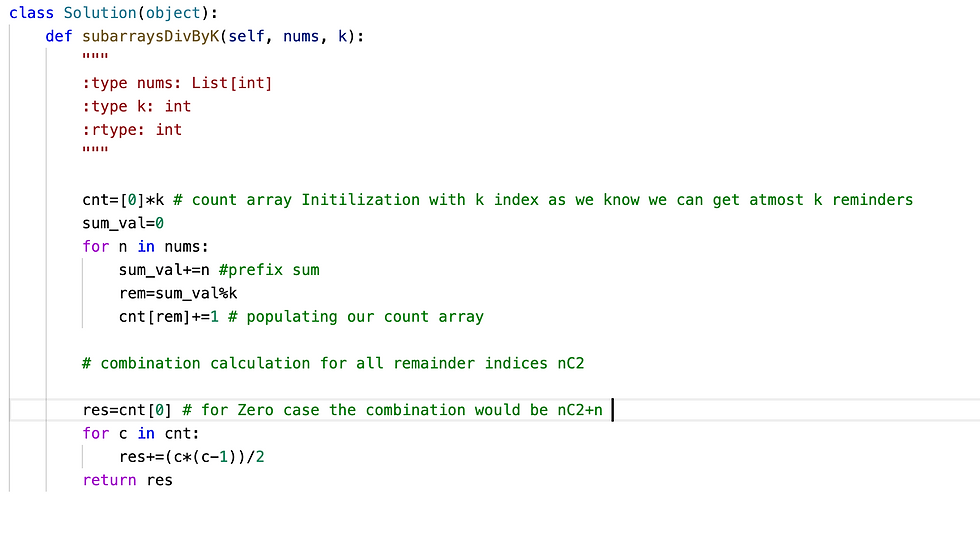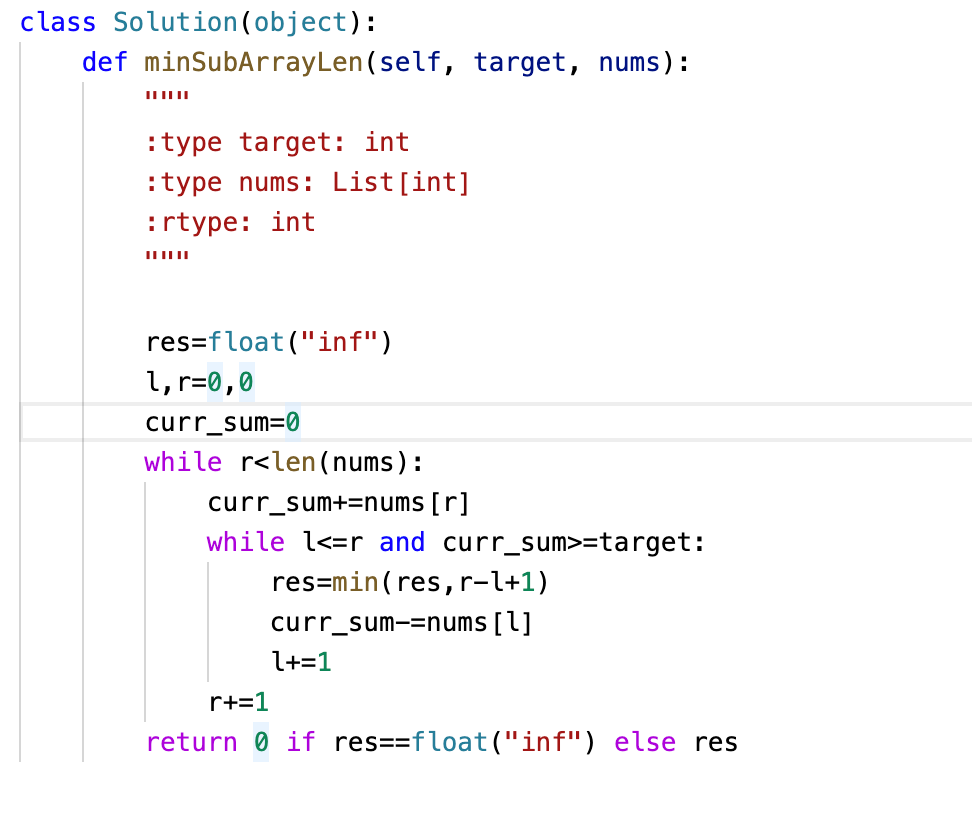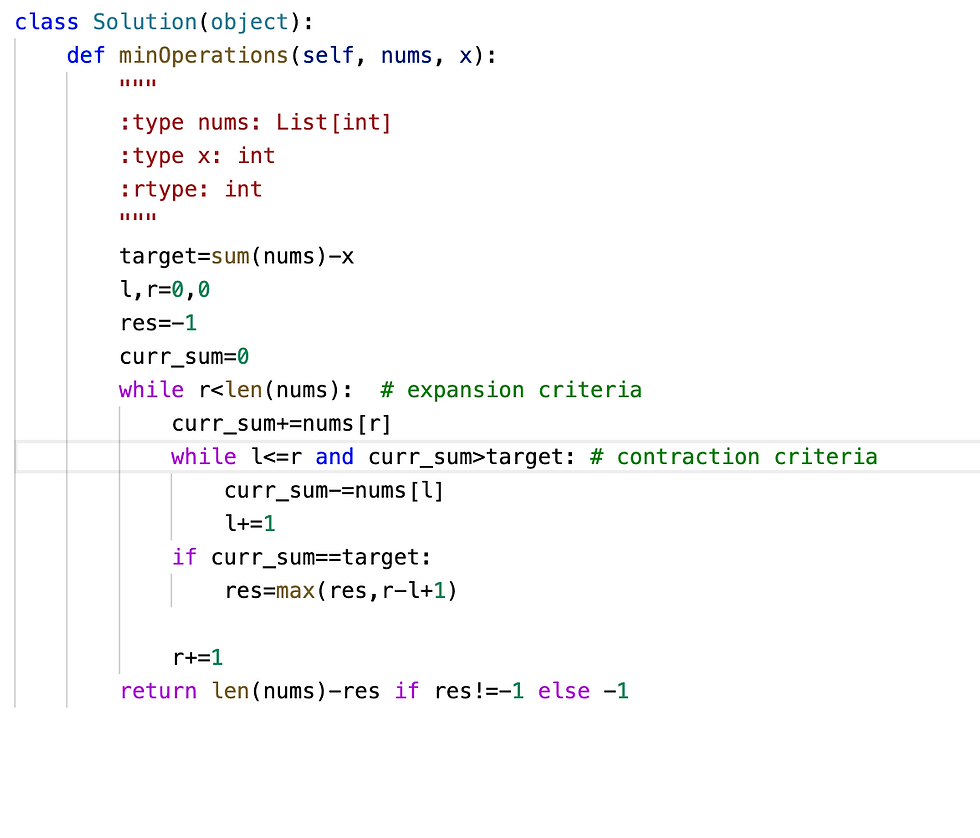top of page
Search

# Sub Arrays Problems LeetCode ~ Top Questions to Practise

Updated: Aug 13Sub Arrays Pattern are repetitive at interviews , Below are few important problems along with solution and it's explanation to get the gist out it.

Question 1: Continuous Subarray Sum

Problem: Given an integer array nums and an integer k, return true if nums has a good subarray or false otherwise.

A good subarray is a subarray where:

• its length is at least two, and

• the sum of the elements of the subarray is a multiple of k.

```Input: nums = [23,2,4,6,7], k = 6
Output: true
Explanation: [2, 4] is a continuous subarray of size 2 whose elements sum up to 6.```

Solution:

Time Complexity O(n)

Memory Complexity O(k)Explanation:

• While Solving it using Brute Force the time complexity would be O(n2) but using below approach problem can be solved with O(n) time complexity.

• Do Prefix Sum Calculation while iterating over nums and store it in HashMap.

• Multiple Prefixes with Same Remainder means that while iterating over we have added extra elements whose sum%k is 0, This indicate that we have found our subarray.

• HashMap initialized with {0:-1} to handle the edge case if first value of nums is divisible by K.

Question 2: Subarray Sums Divisible by K

Problem:Given an integer array nums and an integer k, return the number of non-empty subarrays that have a sum divisible by k.

A subarray is a contiguous part of an array.

```Input: nums = [4,5,0,-2,-3,1], k = 5
Output: 7
Explanation: There are 7 subarrays with a sum divisible by k = 5:
[4, 5, 0, -2, -3, 1], , [5, 0], [5, 0, -2, -3], , [0, -2, -3], [-2, -3]```

Solution:

Time Complexity O(n)

Memory Complexity O(k)Explanation:

The problem is simply extension of above problem but instead of detecting subarray with criteria we simply need to count how many of them are like having subarray sum divisible by k.

• Do Prefix Sum Calculation while iterating over nums and store it in cnt array(each index indicate the remainder of prefix sum %K).

• Multiple Prefixes with Same Remainder means that while iterating over we have added extra elements whose sum%k is 0, This indicate that we have found our subarray. Update the remainder count by 1 every time we have same remainder

• Create combination nC2 for all the remainders except for 0 as we will have combination count as n+nC2

Question 3: Subarray Product Less Than K

Problem: Given an array of integers nums and an integer k, return the number of contiguous subarrays where the product of all the elements in the subarray is strictly less than k.

```Input: nums = [10,5,2,6], k = 100
Output: 8
Explanation: The 8 subarrays that have product less than 100 are:
, , , , [10, 5], [5, 2], [2, 6], [5, 2, 6]
Note that [10, 5, 2] is not included as the product of 100 is not strictly less than k.```

Solution:

Time Complexity O(n)

Memory Complexity O(1)Explanation:

The problem can be solved using two pointer technique with criteria to expand and contract

• The first while loop iterates over nums to expand the subarray with running product

• The nested while loop contacts subarray with reverse criteria running product>=k

• When the contracting criteria is False just sum up the result with current subarray.

Question 4:Subarray Sum Equals K

Problem: Given an array of integers nums and an integer k, return the total number of subarrays whose sum equals to k.

A subarray is a contiguous non-empty sequence of elements within an array.

```Input: nums = [1,1,1], k = 2
Output: 2```

Solution:

Time Complexity O(n)

Memory Complexity O(n)Explanation:

• Initialize HashMap with 0 prefix subarray as count 1 to handle edge case of subarray sum K starting at 0th index of nums

• Populate HashMap with Prefix Sum as Key and value as count

• On every iteration try to seek the PrefixSum - k at hashmap which indicates the number of way we can create subarray with sum k as difference value(PrefixSum - k) indicate how many subarrays we can remove to make current subarray to sum up to k

Question 5: Minimum Size Subarray Sum

Problem: Given an array of positive integers nums and a positive integer target, return the minimal length of a subarray whose sum is greater than or equal to target. If there is no such subarray, return 0 instead.

```Input: target = 7, nums = [2,3,1,2,4,3]
Output: 2
Explanation: The subarray [4,3] has the minimal length under the problem constraint.```

Solution:

Time Complexity O(n)Explanation:

Apply Two pointer technique to find out minimum length of subarray with defined criteria

Question 6: Minimum Operations to Reduce X to Zero

Problem: You are given an integer array nums and an integer x. In one operation, you can either remove the leftmost or the rightmost element from the array nums and subtract its value from x. Note that this modifies the array for future operations.

Return the minimum number of operations to reduce x to exactly 0 if it is possible, otherwise, return -1.

```Input: nums = [1,1,4,2,3], x = 5
Output: 2
Explanation: The optimal solution is to remove the last two elements to reduce x to zero.

```

Solution:

Time Complexity O(n)Explanation:

The goal of the problem can be framed as finding maximum length subarray having sum of (sum(nums)-k), you can visualize it as between portion of subarray excluding region near left and right boundaries , so if maximize this portion the left out portion automatically minimizes to gives us minimum count.

Once goal is clear the rest you can apply two pointer technique to find out result.

Question 7: Contiguous Array

Problem: Given an array of integers nums and an integer k. A continuous subarray is called nice if there are k odd numbers on it.

Return the number of nice sub-arrays.

```Input: nums = [1,1,2,1,1], k = 3
Output: 2
Explanation: The only sub-arrays with 3 odd numbers are [1,1,2,1] and [1,2,1,1].```

Solution:

Time Complexity O(n)

Memory Complexity O(n)Explanation:

Step 1: Convert and map the array to 1 and -1 so that whenever equal number and zeros occur the sum of this subarray would be zero

Step2: Run through the input array and calculate prefix sum and store it in dictionary whenever we encounter two similar prefix sum at two different indices of input array we know that a continuous subarray with sum 0 meaning equal 0's and 1's are present between them and we take max of length scanning through the input array.

Question 8: Count Number of Nice Subarrays

Problem: Given an array of integers nums and an integer k. A continuous subarray is called nice if there are k odd numbers on it.

Return the number of nice sub-arrays.

```Input: nums = [1,1,2,1,1], k = 3
Output: 2
Explanation: The only sub-arrays with 3 odd numbers are [1,1,2,1] and [1,2,1,1].```

Solution:

Time Complexity O(n)

Memory Complexity O(n)Explanation:

Step 1: Map the array to 1 if number are odd and 0 while numbers are even

Step2: Problem is now converted to earlier problem i.e No of subarray with sum==k which we have solved above.

Conclusion:

While Solving Subarray sum or product or mathematical criteria based problem mostly these kind of problem can be solved in O(n) time complexity if you try to use two pointer technique or prefix or running sum. I will add more diverse samples to this post in near future so that we have good coverage for this particular pattern.

18 views1 comment
bottom of page